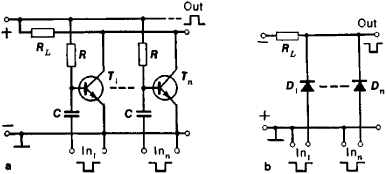# Coincidence Circuit

Also found in: Wikipedia.

## coincidence circuit

[kō′in·sə·dəns ‚sər·kət]
(electronics)
A circuit that produces a specified output pulse only when a specified number or combination of two or more input terminals receives pulses within an assigned time interval. Also known as coincidence counter; coincidence gate.
McGraw-Hill Dictionary of Scientific & Technical Terms, 6E, Copyright © 2003 by The McGraw-Hill Companies, Inc.
The following article is from The Great Soviet Encyclopedia (1979). It might be outdated or ideologically biased.

## Coincidence Circuit

an electronic device that selects from a set of electric-pulse signals received by it only those signals that completely or partially overlap (coincide) in time. It is a discrete-action switching device with two or more inputs and one output. A signal is produced at the output only when signals arrive at all the inputs simultaneously. Such circuits are employed primarily in nuclear electronics, automation, and computer technology.

In nuclear electronics, coincidence circuits are used in conjunction with nuclear radiation detectors. When two or more electric pulses are generated by the detectors in registering individual nuclear-interaction events, it is determined by means of the coincidence circuit whether the pulses are simultaneous. Such coincidence circuits are characterized by three basic parameters: (1) the resolving time Tres, which is the maximum time interval within which input signals are registered as simultaneous, (2) the sensitivity, which is the minimum input signal level (for signals arriving simultaneously at all inputs) at which the circuit operates, and (3) the dead time, which is the minimum time between two successive operations of the coincidence circuit.

Besides the coincidence unit proper, a coincidence circuit includes threshold shaping elements and an output discriminator. The functional elements of coincidence circuits with Tres < 10 nanoseconds are typically combined in a single structural assembly. One method of constructing coincidence circuits is to connect in parallel switching elements (such as transistors or semiconductor diodes) whose internal resistance is small compared with the load resistance (see Figure 1). Coincidence circuits exist that have shaping stages with tunnel diodes. Bridge-type coincidence circuits are also known. The techniques of microelectronics are used to construct matrix coincidence circuits for multiple-input systems. Majority coincidence circuits are coming into use; theyFigure 1. Coincidence circuits: (a) transistor coincidence circuit, (b) diode coincidence circuit; (RL) load resistance, (R) resistor in bias circuit, (C) capacitor in bias circuit, (T1, ..., Tn) transistors, (D1, ..., Dn) diodes

operate when some prescribed number of input signals coincide.

The basic task of coincidence circuits in nuclear physics is to note the occurrence or to establish the number of events that coincide (with complete or partial overlap) within a given time interval. By contrast, coincidence circuits in computers operate when the input signals coincide precisely—that is, overlap completely. In such devices, coincidence circuits perform the functions of, for example, AND logic elements, switches, and switching-matrix elements.